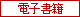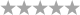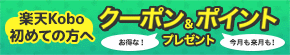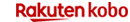トップ > 電子書籍 > 洋書 > Science & Nature > Mathematical Analysis

# Advances in Summability and Approximation Theory　（Springer）　[電子書籍版]

• ユーザ評価0件）
•  | レビューを書く

9,837（税込）## 商品基本情報

• 編集：   S. A. Mohiuddine, Tuncer Acar
• レーベル：  Springer
• 発売日：  2018年12月30日
• 出版社：  Springer Singapore
• 商品番号：  9789811330773
• 言語：  English

## 商品説明

This book discusses the Tauberian conditions under which convergence follows from statistical summability, various linear positive operators, Urysohn-type nonlinear Bernstein operators and also presents the use of Banach sequence spaces in the theory of infinite systems of differential equations. It also includes the generalization of linear positive operators in post-quantum calculus, which is one of the currently active areas of research in approximation theory. Presenting original papers by internationally recognized authors, the book is of interest to a wide range of mathematicians whose research areas include summability and approximation theory.

One of the most active areas of research in summability theory is the concept of statistical convergence, which is a generalization of the familiar and widely investigated concept of convergence of real and complex sequences, and it has been used in Fourier analysis, probability theory, approximation theory and in other branches of mathematics. The theory of approximation deals with how functions can best be approximated with simpler functions. In the study of approximation of functions by linear positive operators, Bernstein polynomials play a highly significant role due to their simple and useful structure. And, during the last few decades, different types of research have been dedicated to improving the rate of convergence and decreasing the error of approximation.

まだレビューがありません。

## 楽天ブックスランキング情報

•週間ランキング

ランキング情報がありません。

•日別ランキング

ランキング情報がありません。電子書籍版

## 最近チェックした商品

※表示を削除したい場合は閲覧履歴のページから削除お願いいたしますこのページの先頭へ